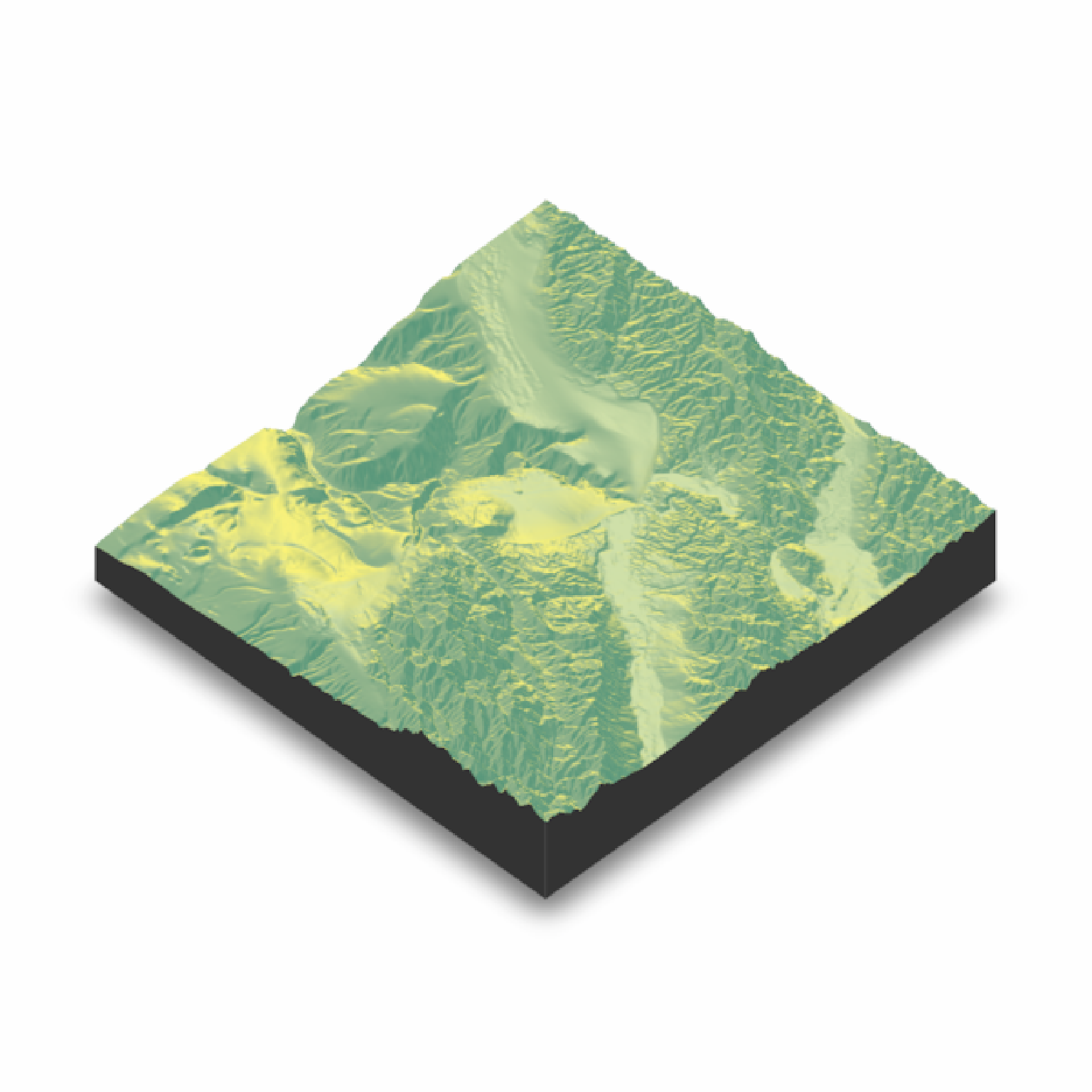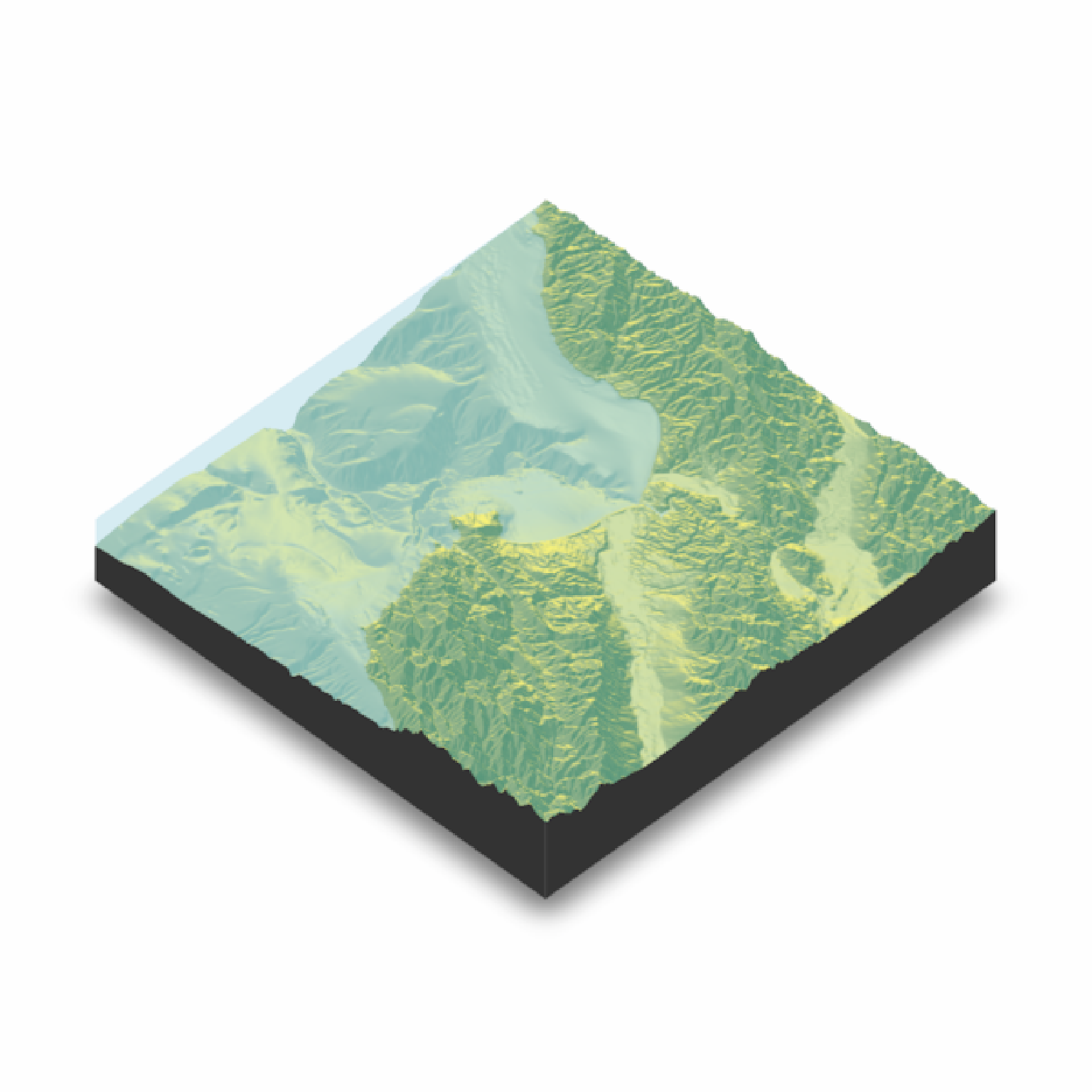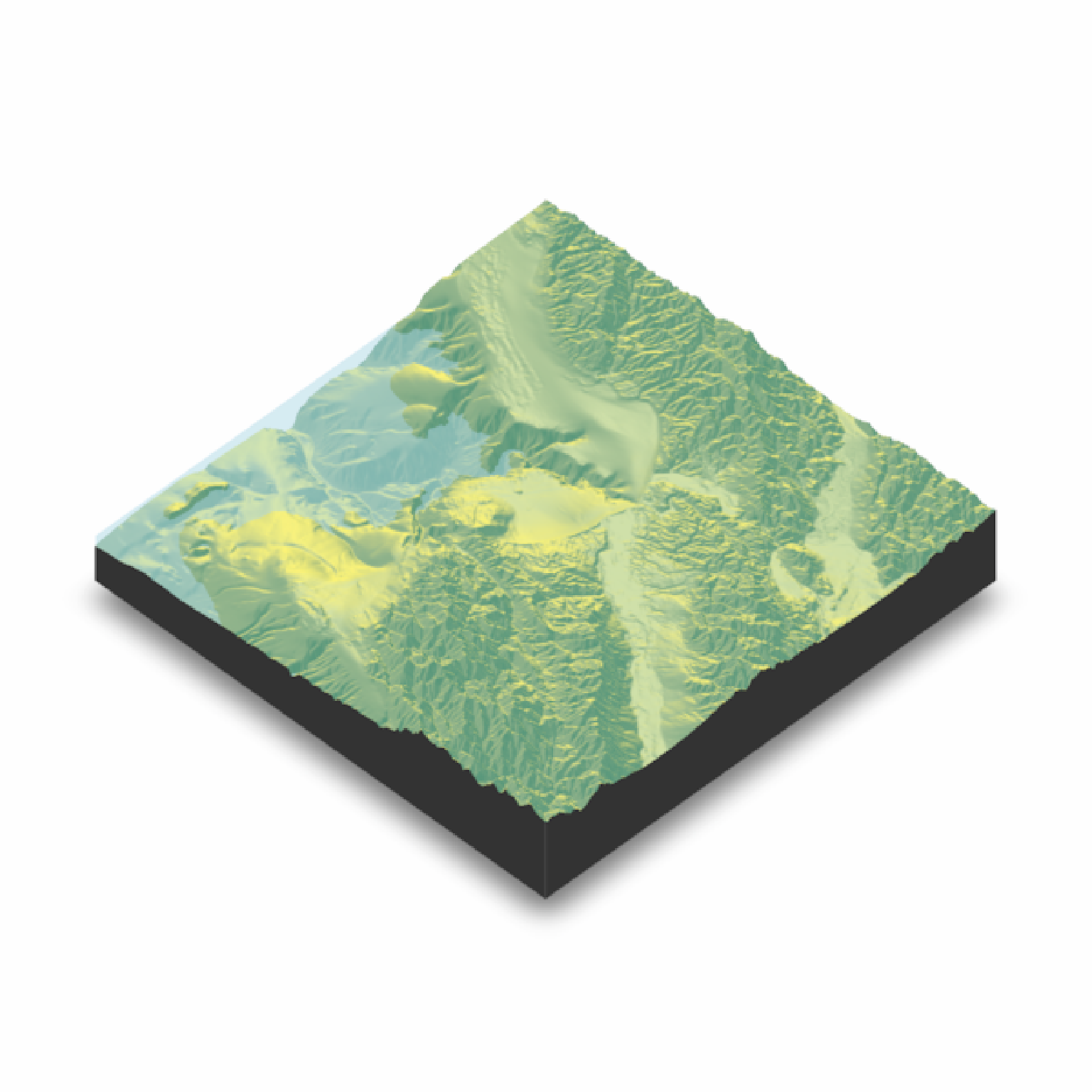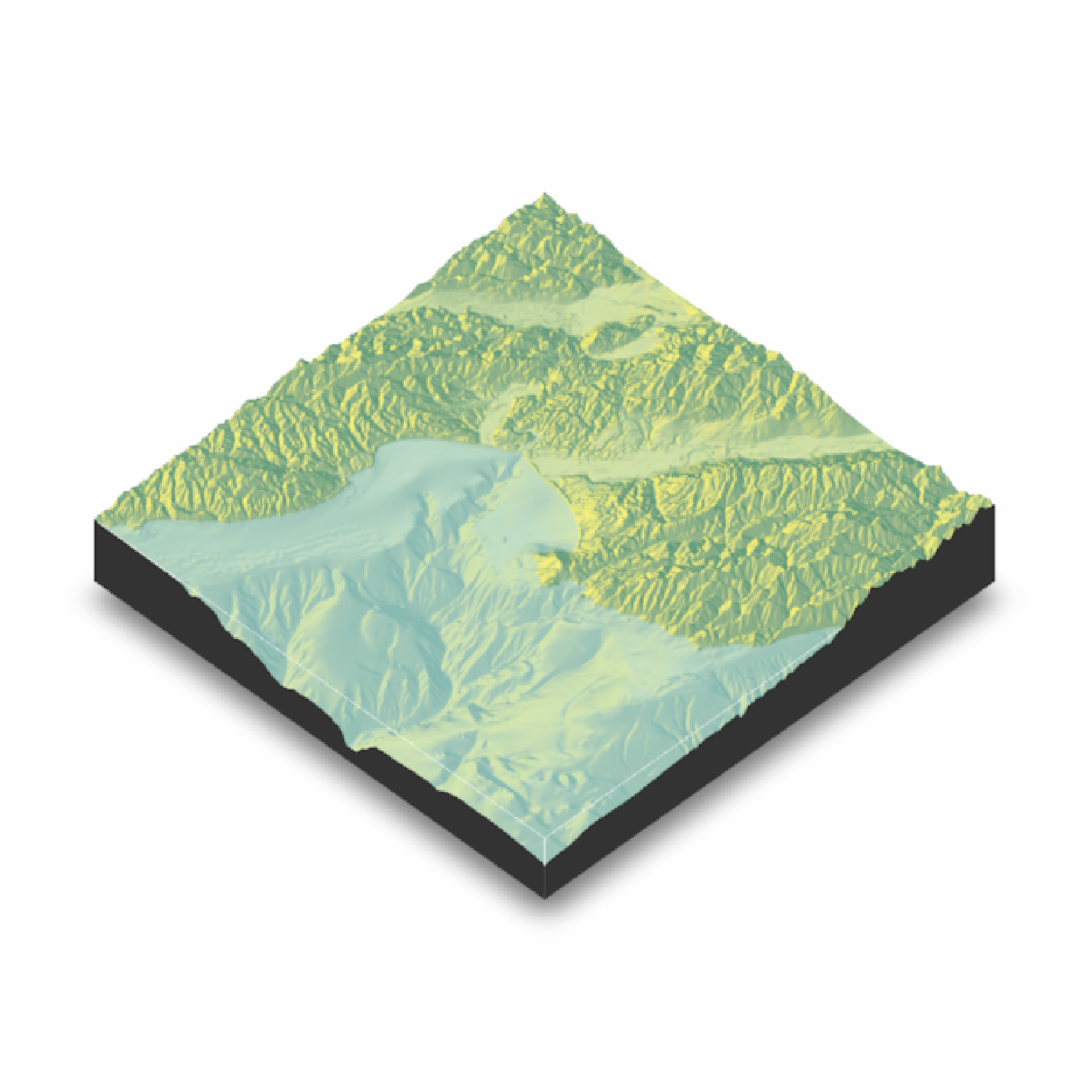Adds water layer to the scene, removing the previous water layer if desired.

render_water(
heightmap,
waterdepth = 0,
watercolor = "lightblue",
zscale = 1,
wateralpha = 0.5,
waterlinecolor = NULL,
waterlinealpha = 1,
linewidth = 2,
remove_water = TRUE
)

## Arguments

heightmap

A two-dimensional matrix, where each entry in the matrix is the elevation at that point. All points are assumed to be evenly spaced.

waterdepth

Default 0.

watercolor

Default lightblue.

zscale

Default 1. The ratio between the x and y spacing (which are assumed to be equal) and the z axis. For example, if the elevation levels are in units of 1 meter and the grid values are separated by 10 meters, zscale would be 10.

wateralpha

Default 0.5. Water transparency.

waterlinecolor

Default NULL. Color of the lines around the edges of the water layer.

waterlinealpha

Default 1. Water line tranparency.

linewidth

Default 2. Width of the edge lines in the scene.

remove_water

Default TRUE. If TRUE, will remove existing water layer and replace it with new layer.

## Examples

if(run_documentation()) {
montereybay %>%
plot_3d(montereybay,zscale=50)
render_snapshot()
}#We want to add a layer of water after the initial render.
if(run_documentation()) {
render_water(montereybay,zscale=50)
render_snapshot()
}#Call it again to change the water depth
if(run_documentation()) {
render_water(montereybay,zscale=50,waterdepth=-1000)
render_snapshot()
}